# Qlik Design Blog

All about product and Qlik solutions: scripting, data modeling, visual design, extensions, best practices, etc.Employee

# Counter Aggregation Functions

Today I am going to blog about five Counter Aggregation Functions that can be used in Qlik Sense and QlikView in both charts expressions and the script.

1. Count()
2. MissingCount()
3. NullCount()
4. NumericCount()
5. TextCount()

Before taking a closer look at how we can use each of these functions, let’s first look at the data set I will use for the examples.  Below is the Excel data I will load.  It is a simple list of fruits, their color and quantity.1. Count()

The Count function is probably one of the most common functions that can be used.  In a chart, Count() aggregates the number of values in each chart dimension.  In the script, Count() returns the number of values aggregated in the expression as defined by a group by clause.

Expression for a chart: Count(Distinct Fruit)

In the script below, Count() will return color and the number of fruits that have that color.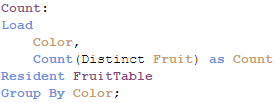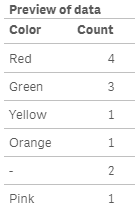2. MissingCount()

In a chart, the MissingCount() function will aggregate the number of missing values in each chart dimension.  In the script, it will return the number of missing values aggregated in the expression, as defined by the group by clause.

Expression for a chart: MissingCount(Quantity)

In the script below, MissingCount() will return 1 if the Quantity field is missing a value.3. NullCount()

NullCount() will return the number of null values in each chart dimension in a chart.  In the script, NullCount() returns the number of null values aggregated in the expression, as defined by a group by clause.

Expression for a chart:  NullCount(Color)

In the script below, NullCount() returns 1 if the Color field is null.4. NumericCount()

In a chart, NumericCount() aggregates the number of numeric values by each chart dimension and in the script, NumericCount() returns the number of numeric values found in the expression, as defined by a group by clause.

Expression for a chart: NumericCount(Quantity)

In the script below, the total Quantity fields that have numeric data is returned.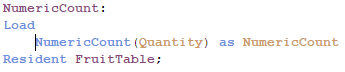5. TextCount()

In a chart, TextCount() aggregates the number of non-numeric values by each chart dimension and in the script, TexCount() returns the number of non-numeric values found in the expression, as defined by a group by clause.

Expression for a chart:  TextCount(Color)

In the script below, TextCount() will return the total number of text values in the Fruit field.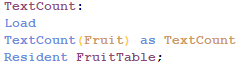Here is a table with all these functions used in a chart: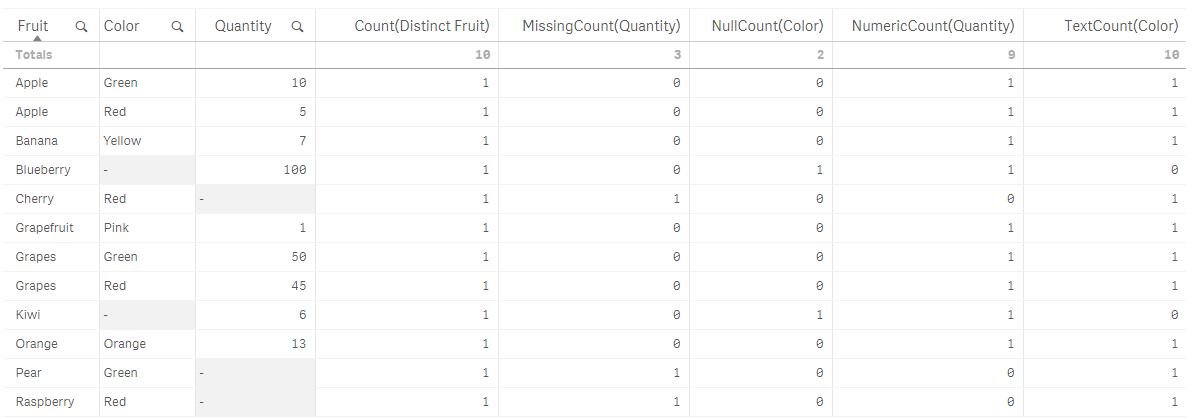While I have not used all of the counter aggregation functions in my work with QlikView and Qlik Sense, I think they can be valuable when auditing and checking the health of your data.  They can highlight gaps in the data that should not be there and point out data type issues in the data.  I would be interested in hearing how you use these functions in your apps.

Thanks,

JennellPartner

What is the difference between functions MissingCount and NullCount?

Or are they just different names for the same function?

248 Views
Contributor III

Clear and good explanations!

248 Views
Contributor III

Hej Anders,

NullCount() will count ONLY the values that are defined as NULL. MissingCount() will count all NULL values and all texts.

br

Paul

248 ViewsPartner

Okay then MissingCount counts non-numeric and null values, find the name a little strange.
'Missing' refers to missing numeric values.

Thus;

MissingCount = NullCount + TextCount

Count = NumericCount + TextCount

and

MissingCount + NumericCount = Count + NullCount

248 ViewsNot applicable

Null count should return the observation with zero values and missing count should return the inexistent observations (they are missing). For instance saying that the income variable is null can mean that you have zero income because you don't work for example and thus do no have a salary. When the income variable has a missing variable it means that it was not observed and can either be null or not. Sometimes the difference comes only from the memory occupied by the observation (null takes no memory while NA does).

Dielia

248 ViewsPartner

Null and zero are not the same thing.
Null is a missing value, zero is an existing value.

Saying the income is null is a statement that you do not know the income, data is missing
Saying the income is zero it is known, data is present.
NullCount should not return observations of zero values and does not.
It is the definition of MissingCount that is a little odd as
the interpretation from Qlik is: Missing Numerical Value.

248 Views
Honored Contributor

Thanks a lot, very useful

248 ViewsNot applicable

You are totally right- my mistake. Indeed null is a missing value- states that the observation does not exist.

Maybe Qlik deals differently with numerical missing values as many software- systems do

248 Views
Valued Contributor

Thanks a lot!

Very clear and good examples.

Regards,

248 Views